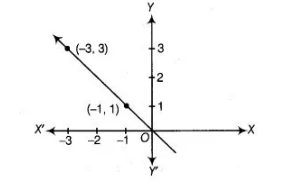# The graph given below represents the linear equation x + y = 0.`
Question:

The graph given below represents the linear equation x + y = 0.Solution:

True

If the given points (-1,1) and (- 3, 3) lie on the linear equation x + y = 0, then both points will satisfy the equation.

So, at point (-1,1), we put x = -1, and y = 1 in LHS of the given equation, we get

LHS = x + y = -1+1 = 0 = RHS

Again, at point (-3 3) put x = – 3 and y = 3 in LHS of the given equation, we get

LHS = x+ y= – 3+ 3= 0 = RHS

Hence, (-1,1) and (-3, 3) both satisfy the given linear equation x + y = 0.